International
Tables for
Crystallography
Volume A
Space-group symmetry
Edited by M. I. Aroyo

International Tables for Crystallography (2015). Vol. A, ch. 1.5, p. 83

Section 1.5.1.3. General change of coordinate system

H. Wondratscheka and M. I. Aroyob

1.5.1.3. General change of coordinate system

| top | pdf |

A general change of the coordinate system involves both an origin shift and a change of the basis. Such a transformation of the coordinate system is described by the matrix–column pair, where the (3 × 3) matrix P relates the new basisto the old one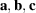according to equation (1.5.1.4). The origin shift is described by the shift vector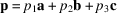. The coordinates of the new originwith respect to the old coordinate systemare given by the (3 × 1) column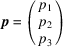.

The general coordinate transformation can be performed in two consecutive steps. Because the origin shift p refers to the old basis, it has to be applied first (as described in Section 1.5.1.1), followed by the change of the basis (cf. Section 1.5.1.2):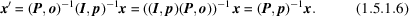Here, I is the three-dimensional unit matrix and o is the (3 × 1) column matrix containing only zeros as coefficients.

The formulae for the change of the point coordinates from x to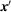uses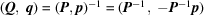, i.e.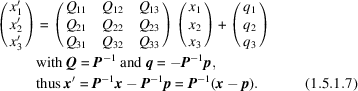The effect of a general change of the coordinate system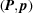on the coefficients of a vector r is reduced to the linear transformation described by P, as the vector coefficients are not affected by the origin shift [cf. equation (1.5.1.3)].

Hereafter, the data for the matrix–column pair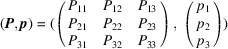are often written in the following concise form: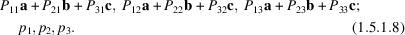The concise notation of the transformation matrices is widely used in the tables of maximal subgroups of space groups in International Tables for Crystallography Volume A1 (2010), wheredescribes the relation between the conventional bases of a group and its maximal subgroups. For example, the expression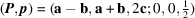(cf. the table of maximal subgroups of, No. 111, in Volume A1) stands for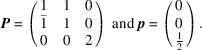Note that the matrix elements of P in equation (1.5.1.8)are read by columns since they act on the row matrices of basis vectors, and not by rows, as in the shorthand notation of symmetry operations which apply to column matrices of coordinates (cf. Section 1.2.2.1).

References

International Tables for Crystallography (2010). Vol. A1, Symmetry Relations between Space Groups. Edited by H. Wondratschek & U. Müller, 2nd ed. Chichester: John Wiley & Sons.